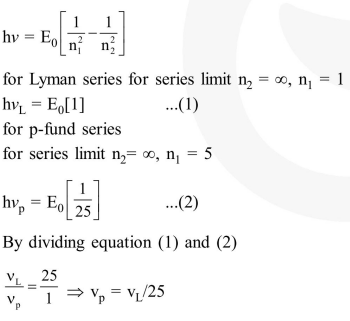# If the series limit frequency of the Lyman series is`
Question:

If the series limit frequency of the Lyman series is $\mathrm{v}_{\mathrm{L}}$, then the series limit frequency of the Pfund series is :

1. (A) $16 \mathrm{v}_{\mathrm{L}}$

2. $v_{L} / 16$

3. $v_{\mathrm{L}} / 25$

4. $25 \mathrm{v}_{\mathrm{L}}$

Correct Option: , 3

Solution: